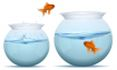LANGUAGE:

# Water and Weighing Puzzles

## Pouring water IIf you had a 5-liter bowl and a 3-liter bowl, and an unlimited access to water, how would you measure exactly 4 liters?

## Pouring water II

Given three bowls: 8, 5 and 3 liters capacity, divide 8 liters in half (4 + 4 liters) with the minimum number of water transfers. Note that the 8-liter bowl is initially filled with 8 liters of water and the other two bowls are empty - that is all water you have.

## Pouring water III

Given three bowls: 7, 4 and 3 liters capacity. Only the 7-liter is full. Pouring the water the fewest number of times, make the quantities of 2, 2, and 3 liters.

## Pouring water IV

How can you measure 6 liters of water using only 4 and 9-liter bowls?

## Pouring water VMeasure exactly 2 liters of water if you have:
1. 4 and 5-liter glass
2. 4 and 3-liter glass

## Pouring water VI

Given three bowls: bowl A (8 liters capacity) filled with 5 liters of water; bowl B (5 liters capacity) filled with 3 liters of water; and bowl C (3 liters capacity) filled with 2 liters of water.
Can you measure exactly 1 liter, by transferring the water only 2 times?

## Weighing IYou have 10 bags with 1000 coins each. In one of the bags, all coins are forgeries. A true coin weighs 1 gram; each counterfeit coin weighs 1.1 gram.
If you have an accurate scale, which you can use only once, how can you identify the bag with the forgeries? And what if you didn't know how many bags contained counterfeit coins?

## Weighing II

A genuine gummy drop bear has a mass of 10 grams, while an imitation gummy drop bear has a mass of 9 grams. Spike has 7 cartons of gummy drop bears, 4 of which contain real gummy drop bears and the others - imitation.
Using a scale only once and the minimum number of gummy drop bears, how can Spike determine which cartons contain real gummy drop bears?

## Weighing III

This puzzle goes a step further from the previous one.
You have eight bags, each of them containing 48 coins. Five of those bags contain only true coins, the rest of them contain fake coins. A fake coin weighs 1 gram less than a real coin. You have an accurate scale, with the precision of up to 1 gram.
Weighing only once and using the minimum number of coins, how can you find the bags containing the fake coins?

## Weighing IVOne of twelve tennis balls is a bit lighter or heavier (you do not know which) than the others. How would you identify this odd ball if you could use an old two-pan balance scale only 3 times?
You can only balance one set of balls against another, so no reference weights and no weight measurements.

## Weighing V

On a Christmas tree there were two blue, two red, and two white balls. All seemed same. However, in each color pair, one ball was heavier. All three lighter balls weighed the same, just like all three heavier balls.
Using a balance scale twice, identify the lighter balls.

## Weighing VI

There are 9 similar balls. Eight of them weigh the same and the ninth is a bit heavier.
How would you identify the heavier ball if you could use a two-pan balance scale only twice?

## Weighing VII

Given 27 table tennis balls, one is heavier than the others.
What is the minimum number of weighings (using a two-pan balance scale) needed to guarantee identifying the heavy one? Of course, the other 26 balls weigh the same.

## Weighing VIII

Suppose that the objects to be weighed may range from 1 to 121 pounds at 1-pound intervals: 1, 2, 3,..., 119, 120 and 121. After placing one such weight on either of two weighing pans of a pair of scales, one or more precalibrated weights are then placed in either or both pans until a balance is achieved, thus determining the weight of the object.
If the relative positions of the lever, fulcrum, and pans may not be changed, and if one may not add to the initial set of precalibrated weights, what is the minimum number of such weights that would be sufficient to bring into balance any of the 121 possible objects?

## Hourglass IHaving 2 sandglasses: one 7-minute and the second one 4-minute, how can you correctly time 9 minutes?

## Hourglass II

A teacher of mathematics used an unconventional method to measure a 15-minute time limit for a test. He just used 7 and 11-minutehourglasses. During the whole time he turned sandglasses only 3 times (turning both hourglasses at once count as one flip).
Explain how the teacher measured 15 minutes.

## Burning Fuses

Your job is to measure 45 minutes, if you have only two cords and matches to light the cords.

1. The two cords are twisted from various materials and so their different segments can burn at different rates.
2. Each cord burns from end to end in exactly one hour.